﻿ Python Tkinter catch the ball game

# Create a 'Catch the Ball' game with Python and Tkinter

## Python Tkinter Canvas and Graphics: Exercise-10 with Solution

Write a Python program that creates a simple game using Tkinter and the Canvas widget. For example, a "Catch the Ball" game where the player catches falling balls using Tkinter. It is a 1 minute game and finally shows the score.

Sample Solution:

Python Code:

``````import tkinter as tk
import random

# Define constants
CANVAS_WIDTH = 300
CANVAS_HEIGHT = 300
BALL_COLORS = ["red", "green", "blue", "orange", "purple"]
BALL_SPEED = 2
NEW_BALL_INTERVAL = 1000  # in milliseconds
GAME_DURATION = 60  # 60 seconds

class CatchTheBallGame:
def __init__(self, root):
self.root = root
self.root.title("Catch the Ball")

# Create Canvas widget
self.canvas = tk.Canvas(self.root, width=CANVAS_WIDTH, height=CANVAS_HEIGHT, bg="white")
self.canvas.pack()

# Initialize score and game end flag
self.score = 0
self.game_over = False

# Create a list to store ball objects
self.balls = []

# Create a Timer to generate new balls at regular intervals
self.root.after(NEW_BALL_INTERVAL, self.create_ball)

# Create a label to display the score and timer
self.info_label = tk.Label(self.root, text="Score: 0 | Time: 60 seconds", font=("Helvetica", 14))
self.info_label.pack()

# Bind mouse click event to the Canvas
self.canvas.bind("<Button-1>", self.catch_ball)

# Initialize the timer
self.timer = GAME_DURATION
self.update_timer()

def create_ball(self):
if not self.game_over:
color = random.choice(BALL_COLORS)
self.balls.append(ball)
self.move_ball(ball)
self.root.after(NEW_BALL_INTERVAL, self.create_ball)

def move_ball(self, ball):
if not self.game_over and ball in self.balls:
self.canvas.move(ball, 0, BALL_SPEED)
x1, y1, x2, y2 = self.canvas.coords(ball)
if y2 > CANVAS_HEIGHT:
self.remove_ball(ball)
self.decrement_score()

# Continue moving the ball
self.root.after(10, self.move_ball, ball)

def remove_ball(self, ball):
if ball in self.balls:
self.balls.remove(ball)
self.canvas.delete(ball)

def catch_ball(self, event):
if not self.game_over:
x, y = event.x, event.y
for ball in self.balls:
x1, y1, x2, y2 = self.canvas.coords(ball)
if x1 < x < x2 and y1 < y < y2:
self.remove_ball(ball)
self.increment_score()

def increment_score(self):
if not self.game_over:
self.score += 1
self.info_label.config(text=f"Score: {self.score} | Time: {self.timer} seconds")

def decrement_score(self):
if not self.game_over and self.score > 0:
self.score -= 1
self.info_label.config(text=f"Score: {self.score} | Time: {self.timer} seconds")

def update_timer(self):
if not self.game_over:
self.timer -= 1
self.info_label.config(text=f"Score: {self.score} | Time: {self.timer} seconds")
if self.timer == 0:
self.end_game()
else:
self.root.after(1000, self.update_timer)

def end_game(self):
self.game_over = True
self.info_label.config(text=f"Game Over | Final Score: {self.score}")

if __name__ == "__main__":
root = tk.Tk()
game = CatchTheBallGame(root)
root.mainloop()
```
```

Explanation:

In the exercise above.

Import the Tkinter library (tkinter).

Constants and configurations are defined:

• 'CANVAS_WIDTH' and 'CANVAS_HEIGHT': Dimensions of the game canvas.
• 'BALL_COLORS': List of possible colors for the balls.
• 'BALL_SPEED': Speed at which the balls fall.
• 'NEW_BALL_INTERVAL': Time interval (in milliseconds) for generating new balls.
• 'GAME_DURATION': Total game duration in seconds.

The 'CatchTheBallGame' class is defined, representing the game:

• It initializes the game window and sets up the canvas, score, and game state.
• It creates a timer to generate new balls at regular intervals.
• It creates a label to display the score and remaining time.
• It binds a mouse click event to the canvas for catching balls.
• It initializes the timer countdown.

The 'create_ball()' method generates a new ball at random positions, assigns a random color, and starts moving it downward.

The 'move_ball()' method updates the position of each ball and checks if a ball has gone out of the canvas. If so, it removes the ball and decreases the score.

The 'remove_ball()' method removes a ball from the canvas and the list of active balls.

The 'catch_ball()' method checks if a click event falls within the bounds of any ball. If so, it removes the ball and increases the score.

The 'increment_score()' and 'decrement_score()' methods update the score label when the score changes.

The 'update_timer()' method updates the timer label and checks if the game time has run out. If the time is up, it ends the game.

The 'end_game()' method sets the game over flag, displays the final score, and prevents further interaction.

The script creates a Tkinter window, initializes the game, and starts the main event loop.

Output:

```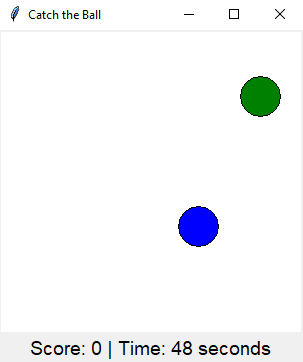```
```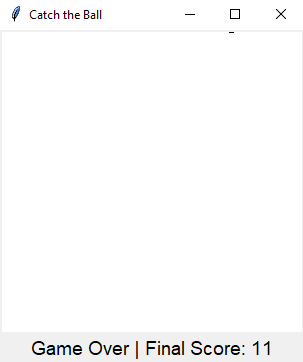```

Flowchart: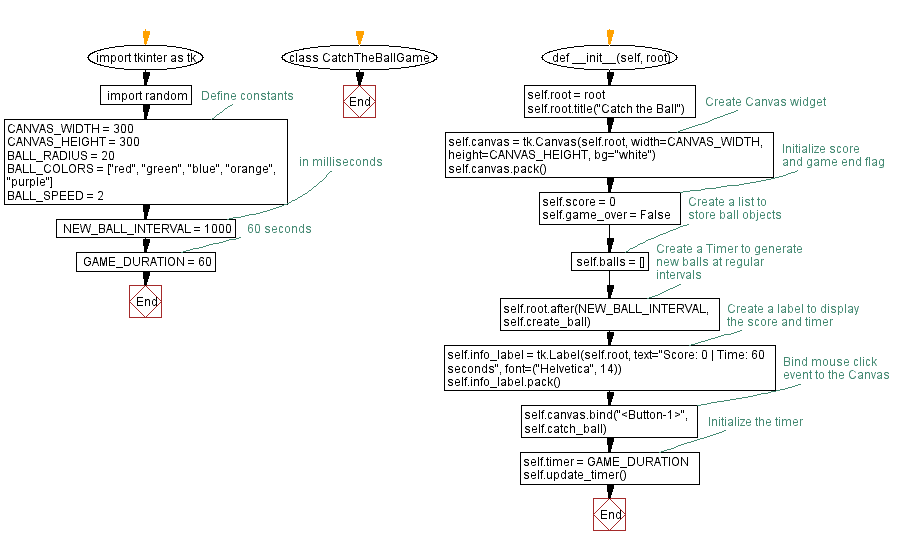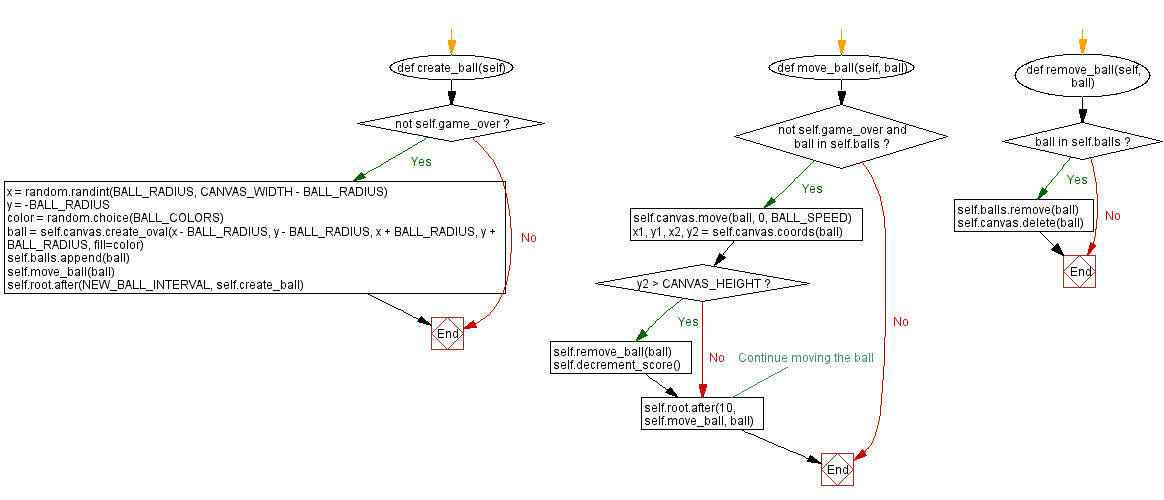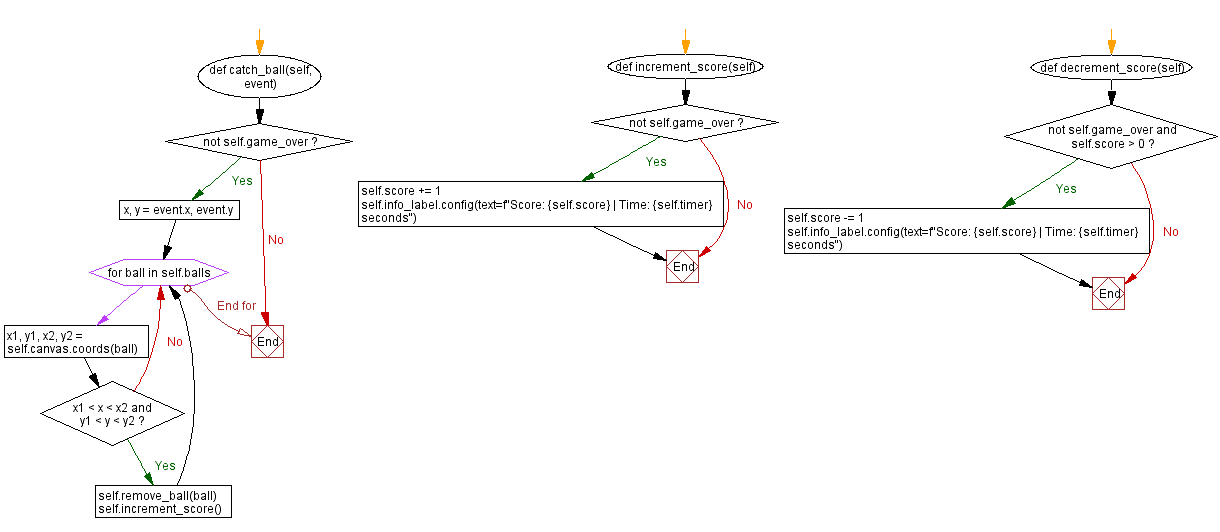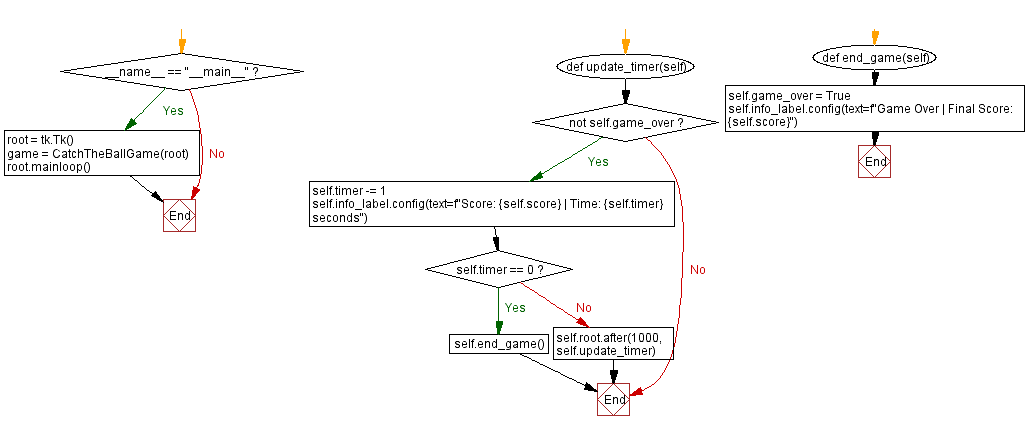Python Code Editor:

What is the difficulty level of this exercise?

Test your Programming skills with w3resource's quiz.

﻿

## Python: Tips of the Day

Summing a sequence of numbers (calculating the sum of zero to ten with skips):

```>>> l = range(0,10,2)
>>> sum(l)
20
```

We are closing our Disqus commenting system for some maintenanace issues. You may write to us at reach[at]yahoo[dot]com or visit us at Facebook